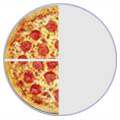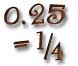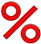# Fractions !

A fraction is a part of a whole

## You can learn about:### Fractions on a pizza and the number line### How to simplify a fraction, and how the "Greatest Common Factor" can help### How to add, subtract, multiply and divide fractions

"Multiplying fractions no big problem,
Top times top over bottom times bottom,

"Dividing fractions, easy as pie,
Flip the second fraction, then multiply,

"If adding or subtracting is your aim,
The bottom numbers must be the same!

"Change the bottom using multiply or divide,
But the same to the top must be applied,

"And don't forget to simplify,
Before it's time to say goodbye"
♫

### How to convert fractions to decimals and vice versa### How to convert fractions to percentages and vice versa